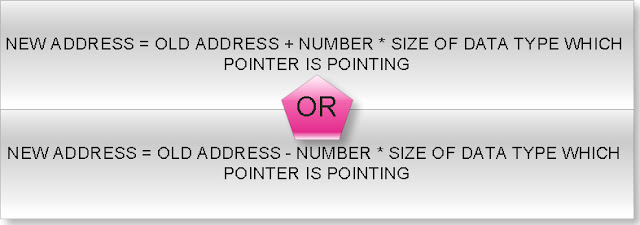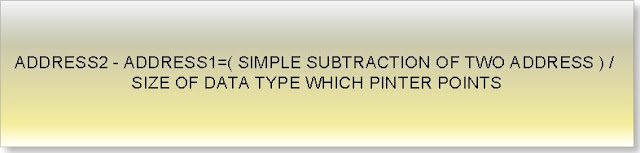## INDEX

### Arithmetic operation with pointer in c programming

Rule 1: Addition arithmetic with pointers

If we will add or subtract a number from an address result will also be an address.(1)What will be output of following c program?

#include<stdio.h>

int main(){

int *ptr=( int *)1000;

ptr=ptr+1;
printf(" %u",ptr);

return 0;
}

Output: 1002

(2)What will be output of following c program?

#include<stdio.h>

int main(){

double *p=(double *)1000;

p=p+3;
printf(" %u",p);

return 0;
}

Output: 1024

(3)What will be output of following c program?

#include<stdio.h>

int main(){

float array={1.1f,2.2f,3.3f};
float(*ptr);

ptr=&array;
printf("%u",ptr);

ptr=ptr+1;
printf(" %u",ptr);

return 0;
}

Output: 1000 1020

(4)What will be output of following c program?

#include<stdio.h>

typedef struct abc{
int far*a;
double b;
unsigned char c;
}ABC;

int main(){

ABC *ptr=(ABC *)1000;

ptr=ptr+2;
printf(" %u",ptr);

return 0;
}

Output: 1026

(5)What will be output of following c program?

#include<stdio.h>

typedef union abc{
char near*a;
long double d;
unsigned int i;
}ABC;

int main(){

ABC *ptr=(ABC *)1000;

ptr=ptr-4;
printf(" %u",ptr);

return 0;
}

Output: 960

(6)What will be output of following c program?

#include<stdio.h>

float * display(int,int);
int max=5;

int main(){

float *(*ptr)(int,int);

ptr=display;

(*ptr)(2,2);
printf("%u",ptr);

ptr=ptr+1;
printf(" %u",ptr);

return 0;
}

float * display(int x,int y){
float f;
f=x+y+max;
return &f;
}

Output: Compiler error

Rule 2: Difference arithmetic with pointers

If you will subtract two pointers result will be a number but number will not simple mathematical subtraction of two addresses but it follow following rule:
If two pointers are of same type then:Consider following example:

#include<stdio.h>

int main(){

int *p=(int *)1000;
int *temp;

temp=p;
p=p+2;

printf("%u %u\n",temp,p);
printf("difference= %d",p-temp);

return 0;
}

Output: 1000 1004
Difference= 2

Explanation:
Here two pointer p and temp are of same type and both are pointing to int data type varaible.
p-temp = (1004-1000)/sizeof(int)
=4/2
=2

(1)What will be output of following c program?

#include<stdio.h>

int main(){

float *p=(float *)1000;
float *q=(float *)2000;

printf("Difference= %d",q-p);

return 0;
}

Output: Difference= 250

Explanation:
q-p=(2000-100)/sizeof(float)
=1000/4
=250

(2)What will be output of following c program?

#include<stdio.h>

struct abc{
signed char c;
short int i;
long double l;
};

int main(){

struct abc *p,*q;

p=(struct abc *)1000;
q=(struct abc *)2000;

printf("Difference= %d",q-p);

return 0;
}

Output: Difference= 76

Explanation:

q-p=(2000-1000)/sizeof(struct abc)
=1000/(1+2+10)
=1000/13
=76

(3)What will be output of following c program?

#include<stdio.h>

typedef union xxx{
char far * c;
const volatile i;
long int l;
}XXX;

int main(){

XXX *p,*q;

p=(XXX *)1000;
q=(XXX *)2000;

printf("Difference= %d",q-p);

return 0;
}

Output: Difference= 250

Explanation:
q-p=(2000-100)/max(4,2,4)
=1000/4
=250

(4)What will be output of following c program?

#include<stdio.h>

int main(){

const volatile array={0};
const volatile(*p)=&array;
const volatile(*q)=&array;

q++;
q++;

printf("%u %u\n",p,q);
printf("Difference= %d",q-p);

return 0;
}

Output: 1000 1016 (assume)
Difference= 2

Explanation:
q-p=(1016-1000)/sizeof(const volatile)
= 16/ (2*4)
=2

Rule 3: Illegal arithmetic with pointers

What will be output of following c program?

#include<stdio.h>

int main(){

int i=5;
int *p=&i;
int *q=(int *)2;

printf("%d",p+q);

return 0;
}

Output: Compiler error

Rule 4: We can use relation operator and condition operator between two pointers.

a. If two pointers are near pointer it will compare only its offset address.

What will be output of following c program?

#include<stdio.h>

int main(){

int near*p=(int near*)0x0A0005555;
int near*q=(int near*)0x0A2115555;

if(p==q)
printf("Equql");
else
printf("Not equal");

return 0;
}

Output: Equal

b. If two pointers are far pointer it will compare both offset and segment address.

What will be output of following c program?

#include<stdio.h>

int main(){

int far*p=(int far*)0x0A0005555;
int far*q=(int far*)0x0A2115555;

if(p==q)
printf("Equql");
else
printf("Not equal");

return 0;
}

Output: Not equal

c. If two pointers are huge pointer it will first normalize into the 20 bit actual physical address and compare to its physical address.

What will be output of following c program?

#include<stdio.h>

int main(){

int huge*p=(int huge*)0x0A0005555;
int huge*q=(int huge*)0x0A2113445;

if(p==q)
printf("Equql");
else
printf("Not equal");

return 0;
}

Output: Equal

Rule 5: Bit wise arithmetic with pointers

We can perform bit wise operation between two pointers like

What will be output of following c program?

#include<stdio.h>

int main(){

int i=5,j=10;
int *p=&i;
int *q=&j;

printf("%d",p|q);

return 0;
}

Output: Compiler error

Rule 6: We can find size of a pointer using sizeof operator.

What will be output of following c program?

#include<stdio.h>

int main(){

int near*far*huge* p;

printf("%d",sizeof(p));
printf(" %d",sizeof(*p));
printf(" %d",sizeof(**p));

return 0;
}

Output: 4 4 2

Definition of pointer
Arithmetic operation with pointer
Pointer to function
Pointer to array of function
Pointer to array of string
Pointer to structure
pointer to union
Multi level pointer
Pointer to array of pointer to string
C tutorial

Mohini said...

pls provide solutions also it would be more helpul and i m having an entrance exam so provide solutions as soon as possible...........

manu said...

void main(){
int huge*p=(int huge*)0x0A0005555;
int huge*q=(int huge*)0x0A2113445;
if(p==q)
printf("Equql");
else
printf("Not equal");

SOUMAVA said...

int *ptr=(int*)1000
here the pointer ptr will point at the integer located at 1000

Sourav said...

In a program i am using 3 types of pointer i.e. int,char,float can these all are accessed by any type of only one pointer in the program.

Satheesh said...

So nice information boss.......Thanks a lot.......

rajiv said...

void main()
{
int far*p=(int far*)0x0a0005555;
int far*q=(int far*)ox0a2115555;
if(p==q)
printf("Equal");
else
printf("Not Equal");
}
plez explain it

rajiv said...

int near*far*huge* p;
plez elaborate this..........

M@NoH@R said...

this is very good

bubbly said...

yeah!!
this is too good...
thanx for sharing this knowledge..

6325655e-e92e-11e0-9ea2-000bcdcb8a73 said...

void main()
{
int far*p=(int far*)0x0a0005555;
int far*q=(int far*)ox0a2115555;
if(p==q)
printf("Equal");
else
printf("Not Equal");
}
Ans:
not equal because we have to check both offset and segment of two pointer.
here though offset is equal in both case but segment part is not equal.
so the ans is "not equal"

ARCHANA said...

thanks allot!

shashi said...

Very good link to understand C pointers

sheetala Prasad soni (JKIE BILASPUR) said...

very easy to understand

ranjith said...

#include

typedef struct abc{
int far*a;
double b;
unsigned char c;
}ABC;

int main(){

ABC *ptr=(ABC *)1000;

ptr=ptr+2;
printf(" %u",ptr);

return 0;
}

Output: 1026

plz explain this program elaborately....
by taking structure padding concept to this...
iam waiting for ur concerned reply...

mousumi said...

Pavan Reddy said...

can u explain me the concept of offset and segment address???

Md. Mahedi Kaysar said...

Swapan said...

Can anyone explain why ques 6 of rule 1 is a compilation error??

hhasini said...

#include

float * display(int,int);
int max=5;

int main(){

float *(*ptr)(int,int);

ptr=display;

(*ptr)(2,2);
printf("%u",ptr);

ptr=ptr+1;
printf(" %u",ptr);

return 0;
}

float * display(int x,int y){
float f;
f=x+y+max;
return &f;
}

Error

Because of this:

ptr=ptr+1;
ptr is a pointer to a function. What do you expect to happen when you add one to a pointer to a function?

Lakshmi natarajan said...